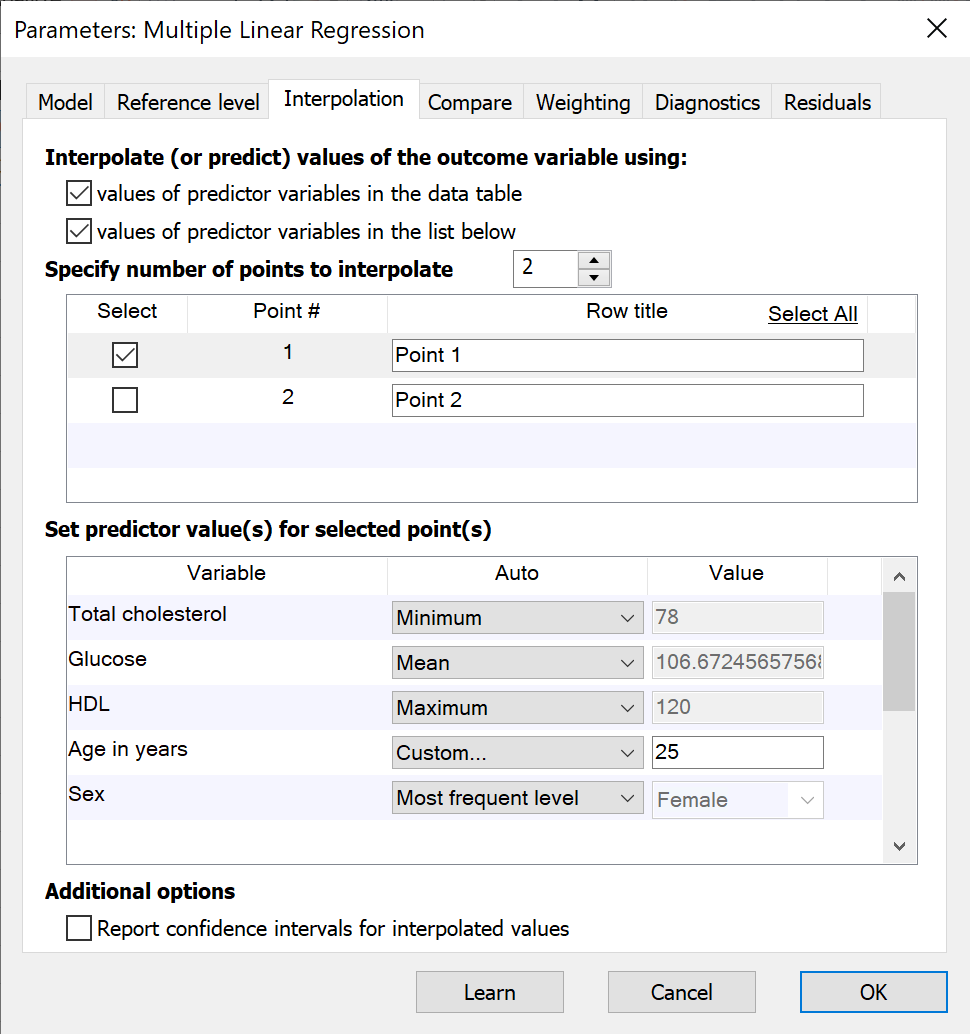## Please enable JavaScript to view this site.

 Interpolation (prediction) with multiple regression

Like simple linear regression and nonlinear regression, Prism also allows for interpolation from multiple linear regression. Using the specified model for multiple regression and the best-fit parameter coefficient, Prism can interpolate values for the outcome variable in two different ways: from points in the input data table and using specified points on the Interpolation tab.## Interpolation of points from the input data table

When this box is checked, Prism will examine the input data table for rows that:

1.Contain a value for each predictor variable in the specified model

2.Do not contain a value for the outcome variable in the specified model

For each of these rows, Prism will use the determined best-fit parameter coefficients along with the the values in the row to calculate the corresponding value for the specified outcome variable. Note that unlike simple linear or nonlinear regression, rows used for interpolation are not required to be at the end of the data table. Prism will search for any row that meets the two criteria above for interpolation.

This feature can be useful when values were recorded for various observations about the predictor variables, but not for the outcome variable

## Interpolation of points from specified list

When this box is checked, Prism allows you to create custom points for interpolation by specifying values for each of the predictor variables. Use the up/down arrows to specify the number of points to be added for interpolation. In the top of the two boxes, a name/label can be added for each interpolated point.

Each point for interpolation must have defined values for each of the predictor variables in the model. To define these values, select an "Auto" method or choose "Custom..." from the dropdown menu. The default settings for each predictor variable are as follows:

Continuous variables: the Auto dropdown menu will be set to "Default" and the value will be zero

Categorical variables: the Auto dropdown menu and value will match the settings of the Reference level for this variable. If the reference values have not been changed manually, the Auto dropdown menu will be set to "First level (default)".

Based on this information, the interpolated value for the outcome variable without changing any of the default values for the predictor variables will be equal to the intercept.

For each predictor variable, you can enter a value or choose  the minimum, maximum, or the value of that variable.

Similarly, for categorical variables, Prism provides options for interpolation using the first level, the last level, or the most/least frequent level in the data. Once again, if the data changes, Prism will automatically update the interpolation accordingly.

Finally, for both continuous and categorical variables, Prism allows for entry of a “Custom…” value to be entered for interpolation.

## Confidence intervals of interpolated values

Prism provides the option to also report the confidence interval of the interpolated value of the outcome variable. To specify the confidence level, use the dropdown menu in the “Calculations” section of the Diagnostics tab.

## What happens to the interpolated (predicted) values if the input data changes?

When the input data for multiple linear regression are changed, Prism will automatically recalculate regression coefficients for the specified model. This will have an effect on the values being interpolated (or predicted) from the model. Additionally, for points interpolated using values of predictor variables listed in the dialog, changes made to the data may change the values of various "Auto" assignment methods.

For continuous variables, Prism provides options to interpolate from the minimum, maximum, or mean value of that variable in the data table (using the "Auto" method dropdown menu). If the data are changed, this minimum, maximum, or mean value may also change, resulting in a different value used during interpolation calculations.

Similarly for categorical variables, Prism provides options to interpolate using the first level, last level, most frequent level, or least frequent level of that variable in the data table (using the "Auto" method dropdown menu). If the data are changed, the first, last, most frequent, or least frequent level may also change, resulting in a different value used during interpolation calculations.

Note that the default "Auto" method (and value) for categorical variables used for interpolation will match the method (and value) of the reference level for that variable. However, once a point is interpolated using a specific method, changing the method for reference level determination will not change the assigned method for the interpolated point.